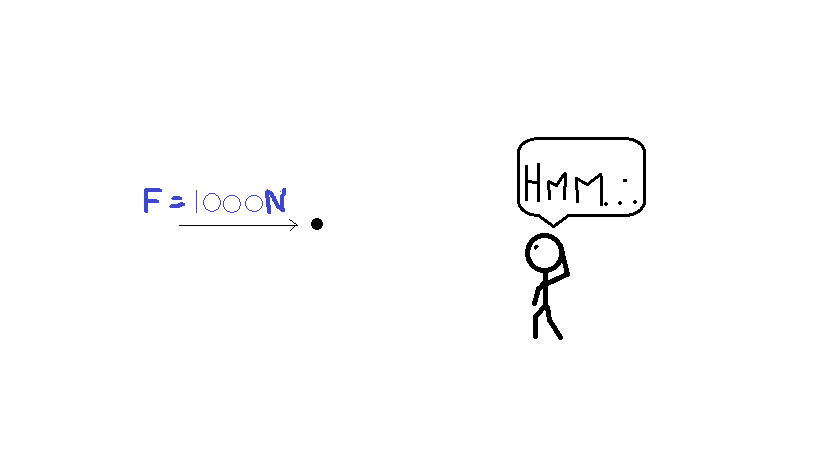# Simple yet complexA particle of mass 1Kg is kept at rest at the origin. A constant force 1000N starts acting on it at t=0. Find the speed of particle at time t=10^6 sec . Let the answer be (a x 10^8) Find a.(Rounding off to 1 decimal place)

×